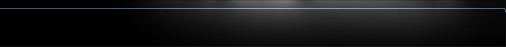//HOME User Manual Glossary Why HIMIPref™ Updates & Notices Contact Us# Yields

## Yield Measures

As described in the Overview HIMIPref™ regards preferred shares as fixed-income instruments and restricts meaningful analysis to those issuers whose strong credit quality supports this assumption. Hence, yield measures must be regarded as the foundation of the analytical system.

### Common Yield Measures

There are two major measures of yield used in most competing forms of analysis, each of which makes certain assumptions which cannot be justified in today's volatile interest rate environment:

• Current Yield : This measure of yield is the simplest form, as discussed in the Glossary. The expected annual dividend rate is divided by the current price of the stock and this measure examined without further analysis. The fatal flaw in this method is the lack of consideration for redemptions and retractions which can lead to unfortunate results. For example, consider two issues: the first is priced at \$27, with an annual dividend of \$2. The second is trading at \$23, with an annual dividend of \$1. The current yields are therefore 7.41% and 4.35% respectively; this method will prefer the former issue. However, potential calls on the issues are ignored completely, that is, they are assigned a probability of exercise of 0%, which is not necessarily the case: if the higher priced issue is redeemed by the issuer at \$25 on the day after purchase, the buyer will realize an immediate capital loss of \$2 and never enjoy any of the benefits of the higher dividend rate. "Current Yield" is a seriously flawed measure.
• Yield-to-Worst : This method examines the options embedded in each issue and assigns an exercise probability of 100% to the option exercise scenario that is most detrimental to the interests of the holder. This method is greatly superior to current yield, as it indicates a floor on the actual return that may be expected by the holder. However, the assignment of a 100% probability to one particular option exercise scenario is flawed - the interest rate environment may change markedly in the time between purchase and potential exercise. Consider these two issues: both are trading at \$24, but one is callable at \$25 while the other is not callable at all. "Yield-to-Worst" will not differentiate between these two issues, because the the call on the former is not "in-the-money", whereas it is obvious that one should prefer the latter, non-callable, issue since there is no cap on the potential capital gains that may be realized should interest rates decline and prices rise. While "Yield-to-Worst" is a better measure than "Current Yield" due to its consideration of at least some embedded options and its conservative assumptions, it should be recognized that the assignment of 100% probability to the worst-case scenario is just as arbitrary, though somewhat less detrimental to the purchaser's interests, as the assumptions implicit in "Current Yield".

### Yield Measures used by HIMIPref™

Accordingly, Hymas Investment Management has developed three entirely new yield measures based on option theory, which provide a far more reliable differentiation of the merits of examined issues:

• Portfolio Yield : In this method, the suite of options that may become exercisable in the future is analyzed to determine the relative probability of each scenario. The cash-flows from each scenario are then analyzed and a standard yield-to-maturity calculation performed on each set. A probability-weighted average is then computed for the instrument as a whole and this figure reported as "Portfolio Yield" - so called because it treats an instrument with embedded options as a portfolio of pseudo-instruments with well-defined maturities.
• Cost Yield : In this method, the same suite of probabilities obtained in the "Portfolio Yield" analysis is analyzed in terms of perturbations of the cash flows expected from the longest-dated pseudo-instrument. Thus, for example, one might say that a particular instrument with a certain term of 10 years, which may be callable in five years, will be treated as a ten-year instrument with a cash-outflow in five years, this cash-outflow representing the probable cost to the investor of the option exercise. Cost is calculated by determining what the expected unrestricted price of the instrument might be and comparing this value with the call price.
• Curve Yield : Slightly different from "Cost Yield" this refinement seeks to value the instruments by determining the cost associated with interim option exercises by determining the cost to the investor of replacing the later-dated presumed cash flow in an interest rate environment which would trigger the exercise of the option.

Differences between the variously defined measures of yields are displayed in the following selection of graphs prepared by HIMIPref™:

 X-Axis Y-Axis Current Yield Yield-to-worst See GRAPH Yield-to-Worst Portfolio Yield See GRAPH Portfolio Yield Cost Yield See GRAPH Cost Yield Curve Yield See GRAPH

The primary design objective of these yield measures was, of course, the determination of a more reliable yield measure than either current yield or yield-to-worst, which has been met. Optimal predictive power is achieved by blending Yield-to-Worst, Portfolio Yield and Cost Yield in almost equal proportions, with a small contribution from Curve Yield. Current Yield has been found to have no practical value when these other measures are available.

Supporting the theoretical utility of the three proprietary measures developed by Hymas Investment Management Inc. for use in HIMIPref is that these measures display the desired property of negative convexity when applied to preferred shares with embedded options. For more details on this property see Analytical Overview - Yield Properties